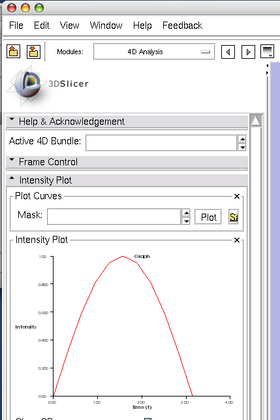# Slicer3:2DPlotting

Home < Slicer3:2DPlotting

## Introduction

Development of 2D plotting in 3D Slicer is in progress as part of 4D Analysis Module Project. The goal is to provide a widget and data structures to plot time series data e.g. time-intensity plot, cardiac signal etc, on 3D Slicer including C++ codes and Python module/console. This page describes the overview of the work and some tutorials.

## Desgin

The 2D plotting is implemented as a set of classes consisting of

• vtkSlicerXYPlotWidget: A widget class to display a graph. This widget observes a plot manager node (vtkMRMLXYPlotManagerNode), and repaints the graph when it receives UpdateGraphEvent from the plot manager node.
• vtkMRMLXYPlotManagerNode: An MRML node class to store graph properties as well as pointers to plot data nodes (vtkMRMLArrayPlotNode).
• vtkMRMLArrayPlotNode: An MRML node class to hold line properties and a pointer to 1D array node (vtkMRMLDoubleArrayNode), which hold array data to be plotted.The diagram of 2D plotting classes.

## Install

Currently, the 2D plotting functionality is part of 4D Analysis Module and you need to build the module manually. Please refer 4D Analysis Module for detailed instruction.

## Tutorial: Plotting Curve from Python Interactor

Here is a small instruction to use the plotting function from Python interface.

0. Open 4D Analysis module in 4D section of the module menu. The XY plot widget is in Intensity Plot Tab.

1. Open Python interactor from "Window" menu.

2. Import packages and get MRML scene:

```>> import Slicer
>> import numpy
>> scene = Slicer.slicer.MRMLScene
```

3. Create a DoubleArray to store a dummy curve data. Suppose we have 11 time points (we need 3 components for each time point to store time, value and standard deviation).

```>> darray = Slicer.slicer.vtkDoubleArray()
>> darray.SetNumberOfComponents(3)
>> darray.SetNumberOfTuples(11)
>> a = darray.ToArray()
```

4. Substitute dummy values into the array.

```>> a[:, 0] = numpy.arange(0, 1.1, 0.1) * numpy.pi
>> a[:, 1] = numpy.sin(a[:, 0])
>> a[:, 2] = 1
```

5. Create an MRML node to hold the array data.

```>> arrayNode = Slicer.slicer.vtkMRMLDoubleArrayNode()
>> arrayNode.SetArray(darray)
```

6. Create an array plot node and set the array node.

```>> arrayPlotNode = Slicer.slicer.vtkMRMLArrayPlotNode()
>> arrayPlotNode.SetAndObserveArray(arrayNode)
```

7. Obtain the plot manager node, which has already been loaded in the 4D Analysis module. We assume that there is only one manager node in 3D Slicer.

```>> pmnode = scene.GetNodeByID('vtkMRMLXYPlotManagerNode1')
```

8. Add the array plot node to the plot manager node.

```>> pmnode.AddPlotNode(arrayPlotNode)
```

9. Refresh the widget.

```>> pmnode.Refresh()
```

After Refresh() function call, the XY plot widget show the sin curve.

10. You my decorate the graph by calling the following functions:

```>> arrayPlotNode.SetColor(1.0, 0.0, 0.0)
>> pmnode.SetXLabel('Time (t)')
>> pmnode.SetYLabel('Intensity')
>> pmnode.Refresh()
```Plot after calling SetColor(), SetXLabel and SetYLabel().